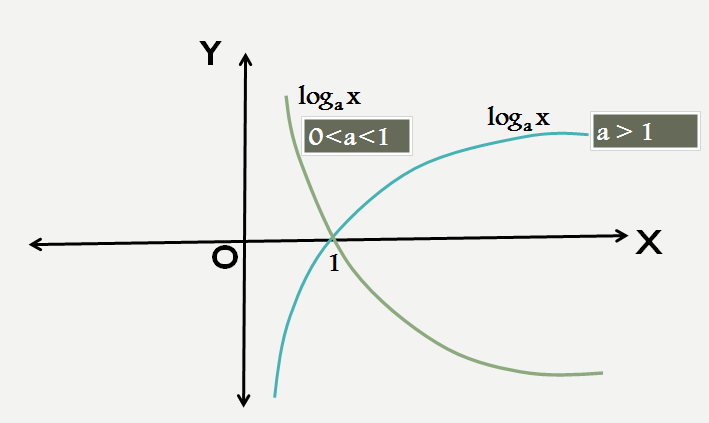## Algebra

#### FUNCTIONS-1

Logarithm: 1

Logarithm is an important chapter in Algebra and is the reverse of the process of exponentiation which raises an exponent of a number. Here logarithm of a number is defined to be the exponent to which a fixed number called as the base must be raised to get that number.

For example, we know that 23 = 8, so the logarithm of 8 with base 2 is 3, i.e. the exponent to which 2 (base) must be raised to generate 8 is 3, written as log28 = 3.

Similarly log4 8 would mean the exponent to which 4(base) must be raised to generate 8 i.e 3/2 or 43/2 = 8

So, in general if ay = x   {Exponential Form}

Then y = logax  {Logarithmic Form}

Here note that x must always be positive while the base (a) must always be positive and its value cannot be equal to 1.

Graph of LogAs one can see that log is not defined for any negative value of x or for x =0. So, one cannot find its curve on the negative X axis. Also, log takes values from  -∞ to +∞. It cuts the X axis at the point (1,0), so log1 = 0 irrespective of the base we are working on.